[ Main page ]

# Analysis of YBC 7289

 The Babylonians used a place system for numbers, with principal base 60, but writing the numbers 0 to 59 in base 10. There were two basic characters, one for 1 and one for 10.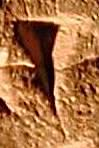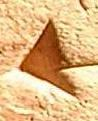1 10
 The rest were assembled from these, making a system more logical if also more verbose than ours. Some of the "digits" are easy to recognize, some more difficult. Particularly difficult are those clustered around the centre of YBC 7289, where the geometrical lines interfere with them. And although in principle the figures other than 1 and 10 were assembled simply from those basic ones, in practice certain patterns were conventionally used which made them easier to decipher.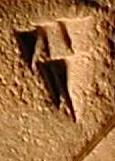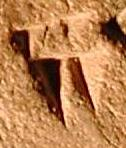2

5

another 5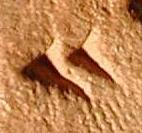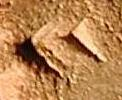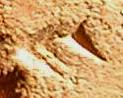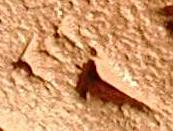20

30 (two views)

40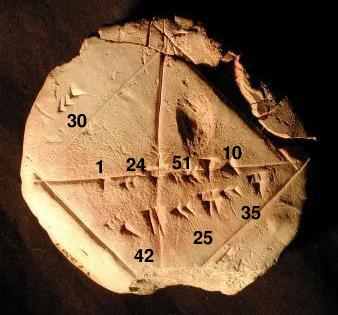Across the diagonal is written 1 24 51 10 = 1 + 24/60 + 51/3600 + 10/216000, which is 1.41421296 to 9 significant decimal figures. The true 9-figure approximation to the square root of 2 is 1.41421356! The 30 at upper left presumably represents a somewhat arbitrary dimension assigned to the side of the square. The length of the diagonal, on this assumption, is 30 times the square root of 2, which is 42 25 35 in base 60.

The point really is that the Babylonians knew that the ratio of diagonal to side was exactly the square root of 2, and they knew how to find a good approximation to that number. You can't get such accuracy with measurements by rulers and a pencil.